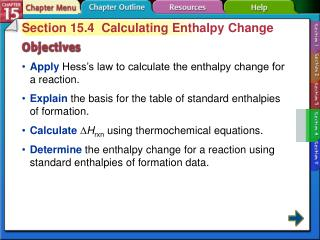DownloadDownload PresentationSection 15-4

# Section 15-4

Download Presentation## Section 15-4

- - - - - - - - - - - - - - - - - - - - - - - - - - - E N D - - - - - - - - - - - - - - - - - - - - - - - - - - -
##### Presentation Transcript

1. Section 15.4 Calculating Enthalpy Change • ApplyHess’s law to calculate the enthalpy change for a reaction. • Explainthe basis for the table of standard enthalpies of formation. • Calculate∆Hrxn using thermochemical equations. • Determinethe enthalpy change for a reaction using standard enthalpies of formation data. Section 15-4

2. Hess's Law • Hess’s lawstates that if you can add two or more thermochemical equations to produce a final equation for a reaction, then the sum of the enthalpy changes for the individual reactions is the enthalpy change for the final reaction. • Sometimes it is impossible or impractical to measure the ∆H of a reaction by using a calorimeter, so Hess’s law allows you to calculate unknown ∆H values using known reactions and their experimentally determined ∆H values Section 15-4

3. Hess's Law (cont.) Section 15-4

4. Standard Enthalpy (Heat) of Formation • The standard enthalpy (heat) of formationis defined as the change in enthalpy that accompanies the formation of one mole of the compound in its standard state from its elements in their standard states. • The standard state of a substance means the normal physical state of the substance at 1 atm and 298 K (25°C). For example, in their standard states, iron is a solid, mercury is a liquid, and oxygen is a diatomic gas. Section 15-4

5. Example: A typical standard heat of formation reaction is the formation of one mole of SO3 from its elements. • S(s) + 3 /2 O 2 (g) → S O 3 (g) ∆ Hf°=-396kJ The product of this equation is SO3

6. Elements in their standard states have a Standard Enthalpy (Heat) of Formation (cont.) • The formation of compounds are placed above or below elements in their standard states. Section 15-4

7. Standard enthalpies of formation of many compounds have been measured experimentally. For example, consider the equation for the formation of nitrogen dioxide. 1 /2N2(g) +O2(g) → NO2(g) ∆Hf° = +33.2 kJ The elements nitrogen and oxygen are diatomic gases in their standard states, so their standard enthalpies of formation are zero. When nitrogen and oxygen gases react to form one mole of nitrogen dioxide, the experimentally determined ∆H for the reaction is +33.2 kJ.

8. That means that 33.2 kJ of energy is absorbed in this endothermic reaction. The energy content of the product NO2 is 33.2 kJ greater than the energy content of the reactants. On a scale on which ∆H f° of reactants is 0.0 kJ, ∆ Hf° of NO2 is +33.2 kJ.

9. Standard Enthalpy (Heat) of Formation (cont.) Section 15-4

10. Standard Enthalpy (Heat) of Formation (cont.) • Standard enthalpies of formation can be used to calculate the enthalpies for many reactions under standard conditions by using Hess’s law. • The summation equation Section 15-4

11. Standard Enthalpy (Heat) of Formation (cont.) Section 15-4

12. Q1/Explain what is meant by Hess’s law and how it is used to determine ∆ H°. Hess’s law says that if two or more equations add up to an overall equation, the ∆H°rxn of the overall equation is the sum of the ∆H° values of the equations that were combined

13. Q2/Describe how the elements in their standard states are defined on the scale of standard enthalpies of formations. • They are assigned enthalpies of formation of zero.

14. A B C D Section 15.4 Assessment What is the enthalpy of oxygen in its standard state? A.0.00 kJ B.15.99 kJ C.100.0 kJ D.8.00 kJ Section 15-4

15. A B C D Section 15.4 Assessment Two or more thermochemical reactions can be summed to determine the overall enthalpy changes based on what law? A.Boyle’s law B.Hess’s law C.Gay-Lussac’s law D.law of conservation of energy Section 15-4

16. A B C D The standard enthalpy of an element in its standard state is ____. A.0.00 calories B.0.00°C C.0.00 kilocalories D.0.00 kJ Chapter Assessment 4

17. End of sec 15.4